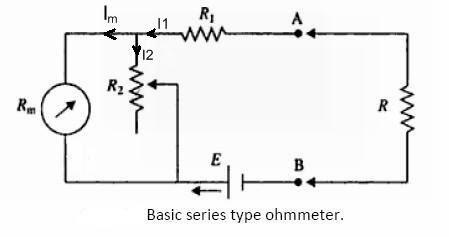# Series type ohmmeter and Shunt type ohmmeter

Hello friends, in this article we are going to learn about Series type ohmmeter along with basic working principle and circuit diagram.

## Ohmmeters

### Introduction:

The ohmmeter is one of the conventional devices which measures the resistance with direct readings.

Ohmmeters has a low degree of accuracy. This does not represent its negative side. Instead, this instrument is widely used in the applications where there is need to determine the approximate value of resistance.

We can use ohmmeters to measure the approximate resistance of electronic components like

• heater elements

• machine field coils

• checking of semiconductor diodes

• checking for continuity of the electic circuit.

Ohmmeters can be used as a precision bridge, it quickly measures the approximate value of resistance which can save a lot of time in balancing the bridge. There are ttwo types of Ohmmetes as 1) Series type ohmmeter and 2) Shunt type ohmmeter. Lets see some more details about these ohmmeters one by one.

### Series type ohmmeter circuit diagram:

Below circuit shows a series tye ohmmter.Basic series type ohmmeter

In the figure,

• Rm is the internal resistance of d’Arsonval movement

• E denotes an emf of internal battery

• R1  is the current limiting resistor

• R2 is a zero adjusting resistor

### Series type ohmmeter working:

In figure, if you can see that a basic d’Arsonval movement  Rm is connected in parallel with a shunting resistor R2. This parallel circuit is connected in series with a battery of emf E and resistance R1. This series circuit is connected to the terminals A and B of resistor Rx. Here Rx is an unknown resistor which we are going to calculate in next steps :).

We know that when any conductor has zero resistance, the current flow will be maximum through that conductor. Similarly, when the unknown resistance Rx is zero (that means there will be no resistor in the circuit, terminals A and B shorted)  maximum current will flow through the meter. For this condition, adjust the resistor R2 so that the basic movement meter indicates full-scale current Ifs. The full-scale current position of the pointer is marked “0” on the scale.

On the other hand, we know that when resistance of a conductor is infinity (that means the two ends of conductor kept open), there will be no flow of current. In this case when Rx is removed from the circuit i.e. Rx =  (that means when terminal A and B are kept open), the current in the meter will be zero and the basic movement indicates zero current which is the marked “”.

In this way our ohmmeter will show infinite resistance at the zero current position and zero resistance at a full scale current position. Here zero resistance indicates that the current in the meter is the maximum and hence the pointer goes to the top mark.

When Rx is inserted at terminal A, B the current through the meter will be less and therefore the pointer will drop lower on the scale. Therefore the meter has “0” at the extreme right and “” at the extreme left position.

Now as we have the range for 0 and infinity resistance on our scale, we can mark intermediate scale by different known values of the resistance Rx to the ohmmeter.

When the pointer of a meter shows the resistance point exactly in the middle of 0 and inifinity, this value of the resistance across terminals A and B is defined as the half scale position resistance Rh.

### You may also like:

Tags: series type ohmmeter derivation, series type ohmmeter ppt, difference between series and shunt type ohmmeter, shunt type ohmmeter wikipedia, difference between series and shunt type ohmmeter pdf, disadvantages of series type ohmmeter, series type and shunt type ohmmeter, multi range ohmmeter

1.Kelly black moffatAugust 26, 2017 at 8:31 AM

2.3.the whole paragraph is copied from book "modern electronic instrumentation and measuring techniques"chapter 4 by william D cooper
we are referring to google because we don't understand book but unluckily for some amount of money you are playing with the students time

4.Thank you

5.Super help full
THANKS

6.Mention in which direction current flows.

7.easy to understanding good

8.